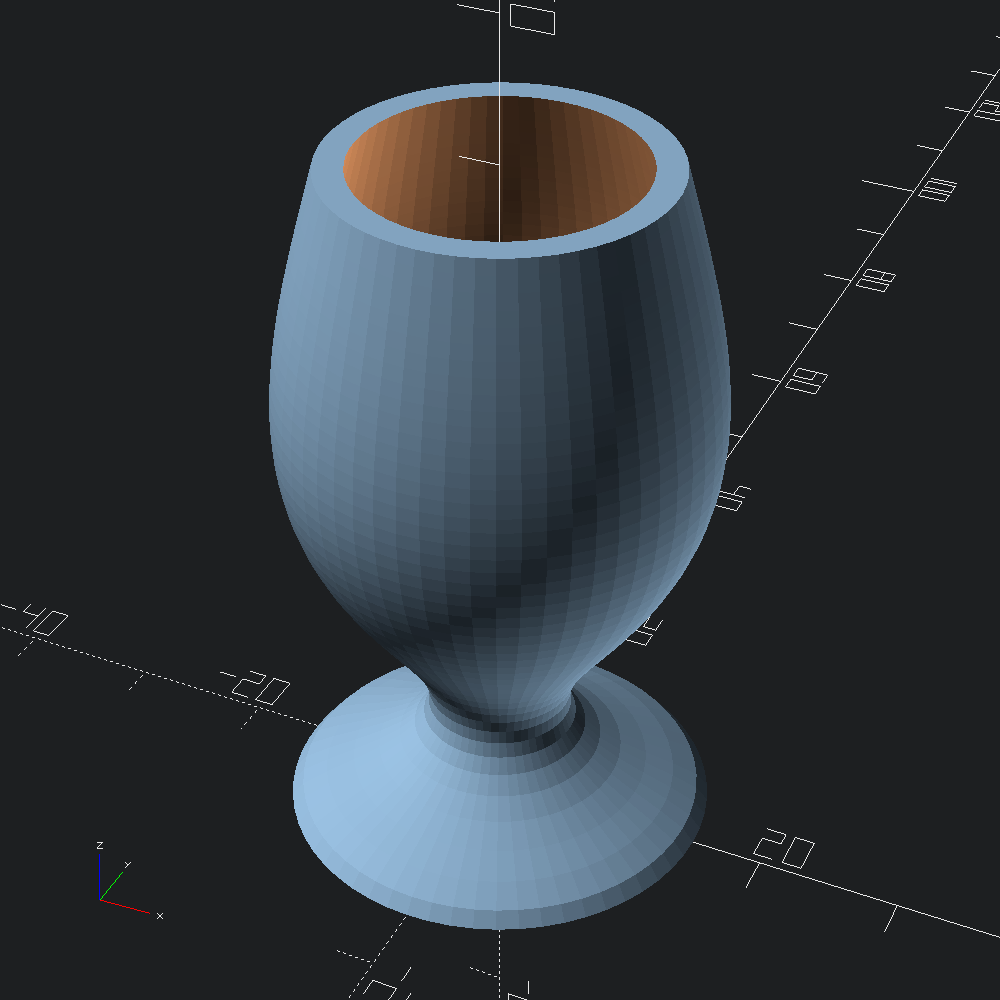# Axial Chalice

``````open OCADml

A function from `z` (angle `a` is ignored) to radius that we will use to define both the outer boundary of the chalice, as well as the cup volume to difference out of it. Since we are ignoring `a`, the radius will be consistent all the way around the z-axis for each height `z`.

``let f ~z ~a:_ = Float.(5. *. (cos (log ((z /. 5.) +. 1.) *. pi) +. 2.))``

Form the `outer` and `inner` (to be subtracted from `outer`) meshes by evaluating `f` between the given z bounds. We'd like `inner` to be narrower that `outer` such that it fits inside, so we subract from the radius returned from `f` uniformly.

``````let outer = Mesh.axial_plot ~min_z:0. ~z_steps:50 ~max_z:50. f
let inner = Mesh.axial_plot ~min_z:2. ~z_steps:50 ~max_z:51. (fun ~z ~a -> f ~z ~a -. 2.)``````

Convert the `outer` and `inner` meshes to `Scad.t`s, and difference them before outputing to file.

``````let () =